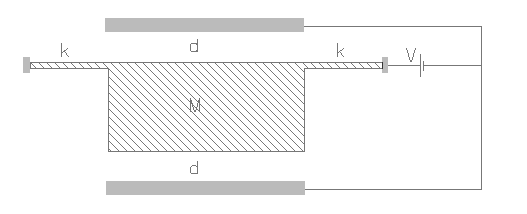ACLSTAT,k,area,m,gap,volt,np,rper,sel
Capacitive accelerometer for sensing a static signal

k            spring constant of the beam in N/m
area      overlapping area of the electrodes in µm2
m           inertial mass in kg
gap       distance between the stationary and movable plate in µm
volt       applied voltage in V
np          number of active stationary plates, 1 plate by default
rper       relative permittivity of the medium, it is approximately 1 for air
sel         number denoting the selected result.
Use 1 for maximum acceleration, 2 for maximum sensitivity and 3 for nonlinearityNotes

This interface could be used for designing a capacitive accelerometer meant to sense a quasi-static signal. It has a mass suspended from a beam with a known spring constant. The stationary electrode can be one or two as shown in the figure above. For capacitance measurement an electrical excitation is applied between the stationary and movable electrodes. This electrical excitation also exerts an electrostatic attraction on the mass which has the movable electrode. The combination of the electrostatic force and the inertial force should not be large enough to send the mass into a pulled-in state where the mass hits one of the stationary electrodes.

This design form can be used to determine the maximum acceleration the device will be able to sense without going into a pull-in state. It also predicts the maximum sensitivity and nonlinearity of the device. The capacitor design or the applied voltage can be changed to increase or decrease the range of operation. The applied voltage usually has a AC component and a DC component typically in the form of ±V1±V2sinωt where frequency ω is much larger than the frequency of the signal and the natural frequency of vibration of the accelerometer. The effective applied voltage can be expressed as

V = √(V12 + V22∕2)

The combination of electrostatic force and inertial force would move the mass to a new stable position. There is a maximum limit to this displacement beyond which the system would be unstable. The acceleration corresponding to that maximum displacement is the maximum acceleration that can be sensed. For a single sided excitation, the total displacement is the sum of displacement due to  electrostatic and inertial forces. But for double sided excitation, the displacement due to electrostatic force is nil because it is canceled by the presence of two electrodes. This allows the the sensing of a larger acceleration load by accelerometer operating with double sided excitation. The maximum sensitivity is calculated as the difference in capacitance before and after the application of the sensing signal assuming the limiting acceleration load is applied to the sensor. For this the initial and final displacement before and after application of the inertial signal is calculated and the equivalent change in capacitance per unit change in acceleration is estimated. This change in capacitance is related to an equivalent change in voltage by the driver electronics. The excitation voltage introduces a nonlinearity term in the acceleration vs displacement function. The estimated nonlinearity corresponds to the maximum operating range. At larger voltages, the sensitivity is higher, but the maximum acceleration that can be sensed would decrease.

The plot is acceleration measured in terms of acceleration due to gravity g versus the displacement of the movable electrode. The plot shows that for a given capacitor design, excitation voltage and acceleration load, there is only one stable solution for displacement. The peak point in the curve is the maximum acceleration beyond which there is no stable solution. The corresponding displacement is the maximum stable displacement. If an acceleration above the maximum limit is applied it will go into a pulled-in state. It can also be seen that for double sided excitation, the operational range is larger than for single sided excitation. Larger reliable operational range would mean a lower sensitivity and hence is a trade-off.

Assumptions

-Static acceleration signal normal to the plane of the mass is assumed.
-Damping effects are not considered.
-The stationary electrodes are arranged symmetrically on both sides of the movable electrode.
-The spring constant of the beam is known. If unknown, this can be calculated under Mechanics > Structures > Beams.
-The medium between the electrodes is air by default with a relative permittivity of 1.
-The gap between the electrodes is uniform.
-The supply voltage is constant and there is no overshooting of the mass.
-The static displacement of the mass before the application of voltage and acceleration signal is considered to be zero.
-Fringe effect on capacitance is not considered